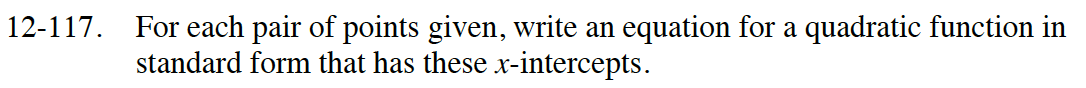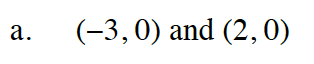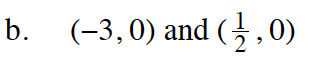### Home > INT2 > Chapter 12 > Lesson 12.2.3 > Problem12-117

12-117.
1. For each pair of points given, write an equation for a quadratic function in standard form that has these x-intercepts. Homework Help ✎

2.  a. (−3, 0) and (2, 0) b. (−3, 0) and (, 0)Work backwards: use the zero product property to write an equation in factored form, then simplify.

x = −3 or x = 2
x + 3 = 0 or x − 2 = 0
(x + 3)(x − 2) = 0
x² + x − 6 = ySee part (a).

$\text{Since we don't usually factor with fractions,}$

$\left(x - \frac{1}{2}\right)\text{ is not considered a factor.}$

$\text{Consider what you would have done to get } \frac{1}{2}.$

$\text{If }x = \frac{1}{2},\text{ the step before that was } 2x = 2.$